## 1. 概述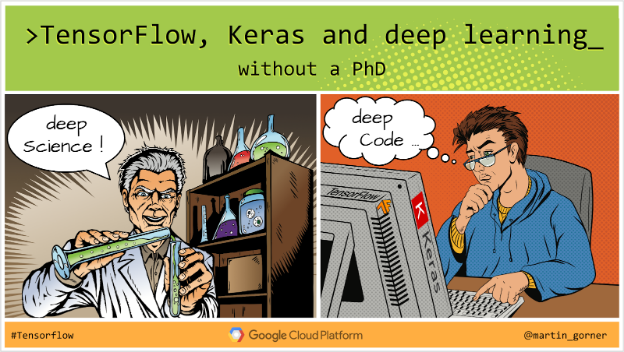• 什么是神经网络(neural network)以及如何训练它
• 如何使用tf.keras构建基本的1层神经网络
• 如何添加更多的神经网络层
• 如何设置学习率(learning rate)时间表
• 如何建立卷积神经网络(convolutional neural networks)
• 如何使用正则化(regularisation)技术：dropout, batch normalization
• 什么是过度拟合(overfitting)

Welcome to Colab.ipynb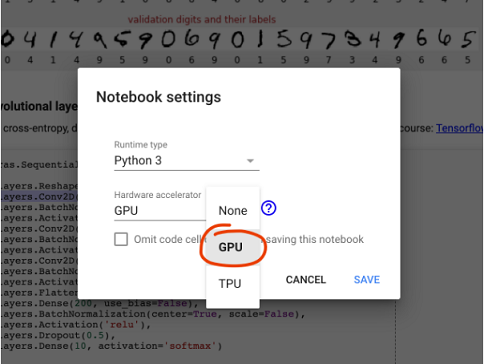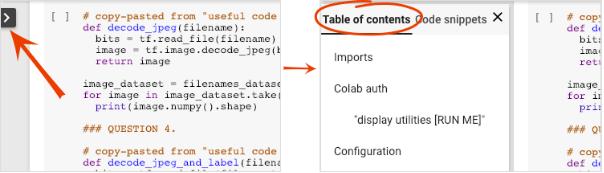## 3. 训练神经网络

keras_01_mnist.ipynb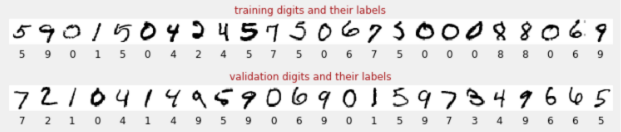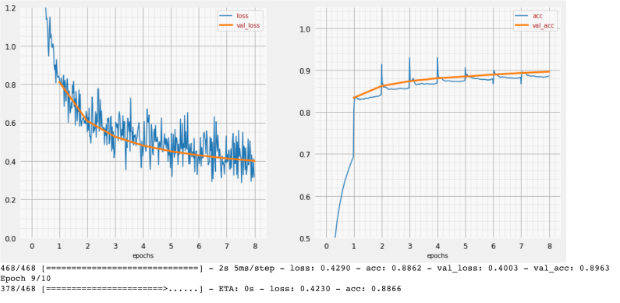X轴表示通过整个数据集的“时代”(epoch)次数或迭代次数。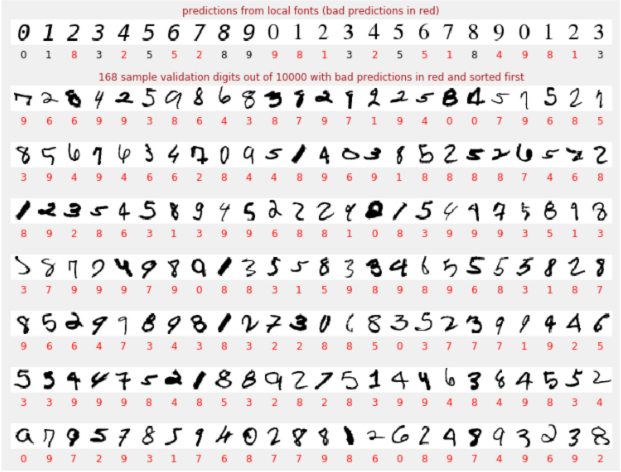## 4. [INFO]：神经网络1011 2 3 4 5 6 7 8 9 10 11 12 13 14 15  model = tf.keras.Sequential([ tf.keras.layers.Flatten(input_shape=[28, 28, 1]), tf.keras.layers.Dense(200, activation="relu"), tf.keras.layers.Dense(60, activation="relu"), tf.keras.layers.Dense(10, activation='softmax') # classifying into 10 classes ]) # this configures the training of the model. Keras calls it "compiling" the model. model.compile( optimizer='adam', loss='categorical_crossentropy', metrics=['accuracy']) # % of correct answers # train the model model.fit(dataset, ... )MNIST数据集中的手写数字是2828像素的灰度图像。对它们进行分类的最简单方法是使用2828=784像素作为1层神经网络的输入。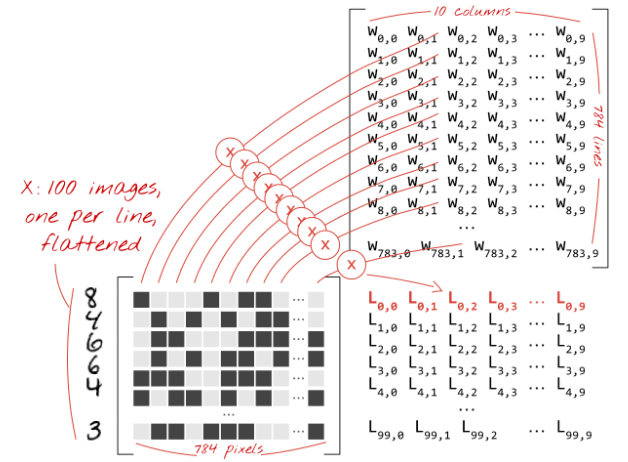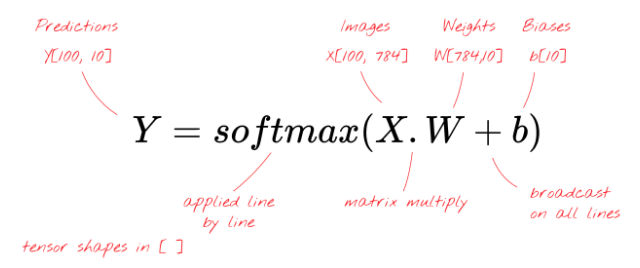Keras

 1  tf.keras.layers.Dense(10, activation='softmax')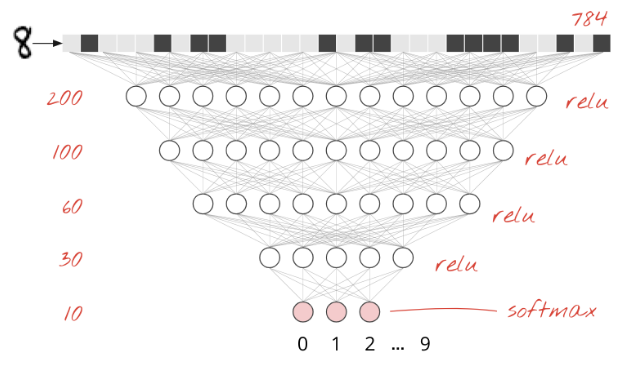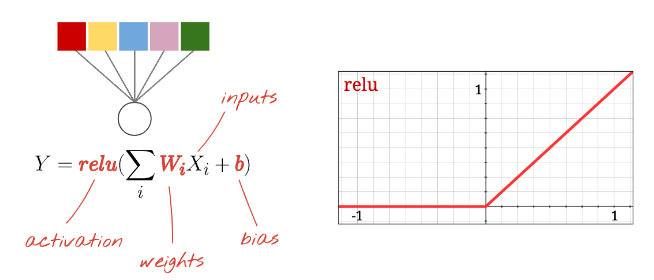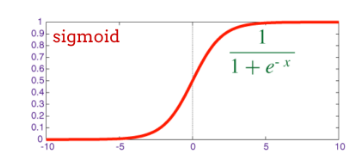Softmax激活函数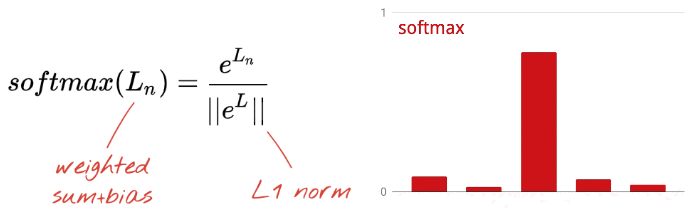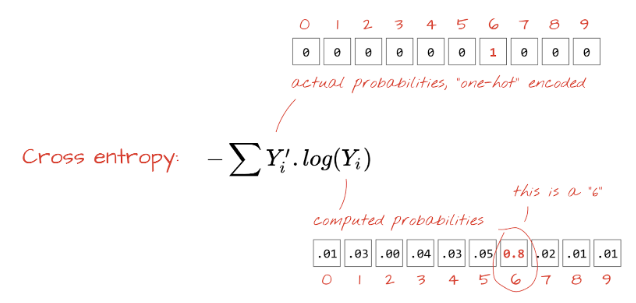“一位有效”(one-hot)编码意味着你使用10个值的向量表示标签“this is digit 3”，除了第3个值为1之外，所有的值都为零，这个向量表示是“digit 3”的概率为100%。我们的神经网络还将其预测结果输出为10个概率值的向量。它们很容易比较。

“训练”神经网络实际上是指使用训练图像和标签来调整权重和偏差，从而最小化交叉熵损失函数。下面是它的工作原理。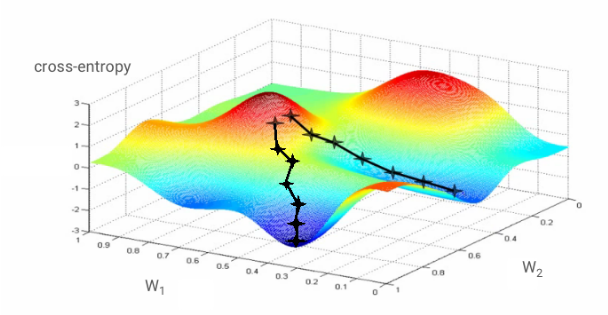“学习率”(Learning rate)：你不能在每次迭代时通过梯度的整个长度来更新权重和偏差。这就像穿着靴子试图走入谷底，你会从山谷的一侧跳到另一侧，要到达底部，你需要做一些小的步骤，即只使用梯度的一小部分，通常在1/1000范围内，这个分数称为“学习率”。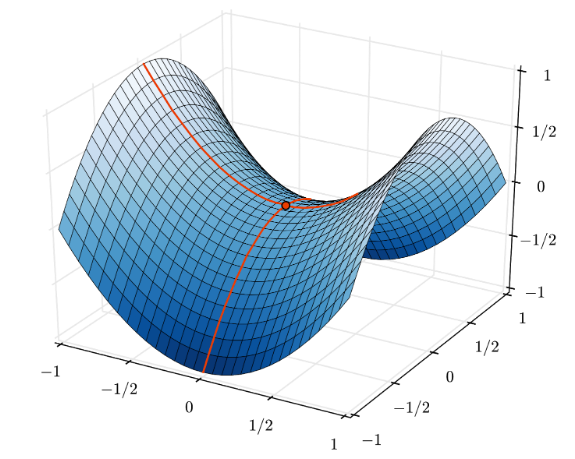TensorFlow库提供了以tf.train.GradientDescentOptimizer开头的全部优化器。具有内置动量的更高级的常用优化器是tf.train.RMSPropOptimizertf.train.AdamOptimizer

logits：在应用激活函数之前，一层神经元的输出称为“logits”。该术语来自“逻辑函数”，又称“Sigmoid函数”，它曾经是最常用的激活函数。“逻辑函数前的神经元输出”简称为“logits”。

relu：修正线性单元。神经元的常用激活函数。

sigmoid：另一个常用的激活函数，在特殊情况下仍然有用。

softmax：一种特殊的激活函数，作用于向量，增加最大部分与所有其他部分之间的差异，并将该向量归一化为总和是1，以便可以将其解释为概率向量。用作分类器的最后一步。

## 5. 让我们看看代码

keras_01_mnist.ipynb

“参数”

“导入”

“可视化实用程序[RUN ME]”

“tf.data.Dataset：解析文件并准备训练和验证的数据集”

MNIST数据集中的图像和标签（正确答案）以固定长度存储在4个文件中，可以使用专用的函数加载：

 1  imagedataset = tf.data.FixedLengthRecordDataset(image_filename, 28*28, header_bytes=16) 

 1 2 3 4 5  def read_image(tf_bytestring): image = tf.decode_raw(tf_bytestring, tf.uint8) image = tf.cast(image, tf.float32)/256.0 image = tf.reshape(image, [28*28]) return image 

 1  imagedataset = imagedataset.map(read_image, num_parallel_calls=16) 

 1  dataset = tf.data.Dataset.zip((imagedataset, labelsdataset)) 

 1 2 3 4 5  dataset = dataset.cache() dataset = dataset.shuffle(5000, reshuffle_each_iteration=True) dataset = dataset.repeat() dataset = dataset.batch(batch_size) dataset = dataset.prefetch(tf.data.experimental.AUTOTUNE) 

tf.data.Dataset API具有准备数据集所需的所有实用函数：

.cache将数据集缓存在内存中。这是一个很小的数据集，因此可以正常工作。.shuffle用5000个元素的缓冲区对其进行轮换。重要的是，对训练数据进行充分的轮换。.repeat重复循环数据集。我们将对其进行多时代的训练(multiple epochs)。.batch将多个图像和标签组成一个批量。最后，.prefetch可以在GPU上训练当前批量数据时，使用CPU准备下一批量数据。

“Keras模型”

Keras模型还需要知道其输入的形态，可以用tf.keras.layers.Input定义它。这里输入的向量是28*28像素的平面向量。

  1 2 3 4 5 6 7 8 9 10 11 12 13 14 15  model = tf.keras.Sequential( [ tf.keras.layers.Input(shape=(28*28,)), tf.keras.layers.Dense(10, activation='softmax') ]) model.compile(optimizer='sgd', loss='categorical_crossentropy', metrics=['accuracy']) # print model layers model.summary() # utility callback that displays training curves plot_training = PlotTraining(sample_rate=10, zoom=1) 

Keras提供了一个非常好的model.summary()函数，可以打印你创建的模型的详细信息，并且还添加了PlotTraining函数（在“可视化实用程序”单元格中定义的），该函数将在训练期间显示各种训练曲线。

“训练和验证模型”

 1 2 3  model.fit(training_dataset, steps_per_epoch=steps_per_epoch, epochs=EPOCHS, validation_data=validation_dataset, validation_steps=1, callbacks=[plot_training]) 

“可视化预测”

 1 2  probabilities = model.predict(font_digits, steps=1) predicted_labels = np.argmax(probabilities, axis=1)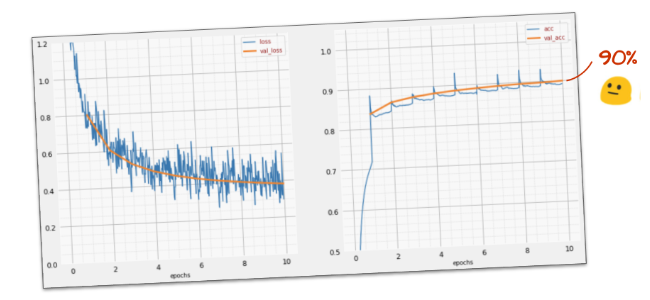## 6. 添加网络层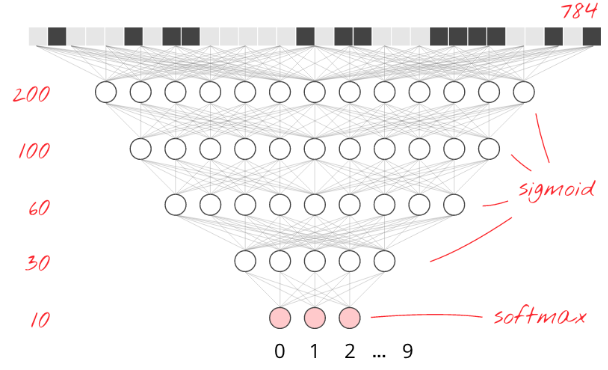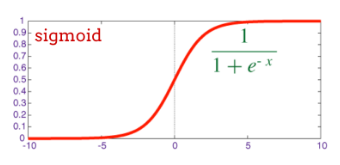1 2 3 4 5 6 7  model = tf.keras.Sequential( [ tf.keras.layers.Input(shape=(28*28,)), tf.keras.layers.Dense(200, activation='sigmoid'), tf.keras.layers.Dense(60, activation='sigmoid'), tf.keras.layers.Dense(10, activation='softmax') ])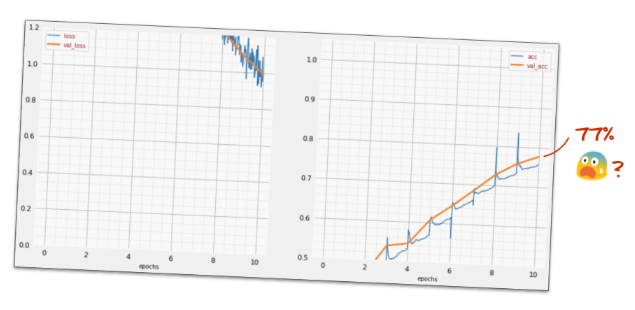## 7. 对深度网络的特别关注

RELU激活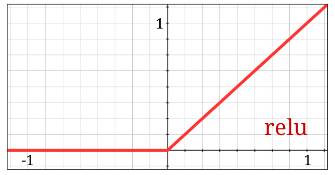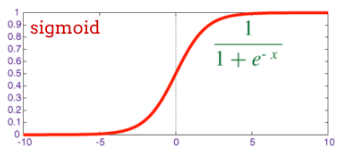NaN ???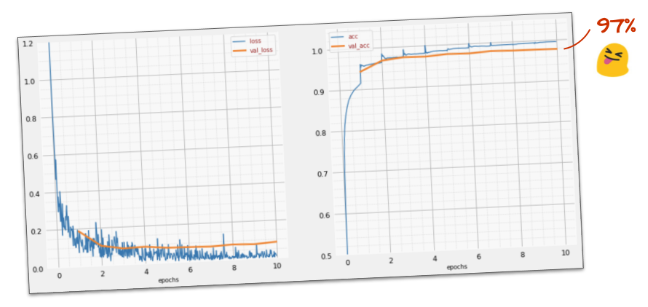keras_02_mnist_dense.ipynb

## 8. 学习率衰减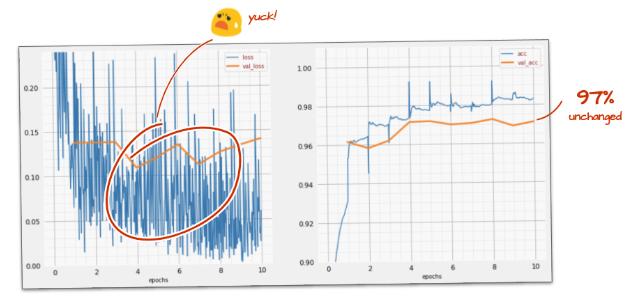1 2 3 4 5 6 7 8 9  # lr decay function def lr_decay(epoch): return 0.01 * math.pow(0.6, epoch) # lr schedule callback lr_decay_callback = tf.keras.callbacks.LearningRateScheduler(lr_decay, verbose=True) # important to see what you are doing plot_learning_rate(lr_decay, EPOCHS) 

 1  model.fit(..., callbacks=[plot_training, lr_decay_callback]) 

## 9. Dropout, overfitting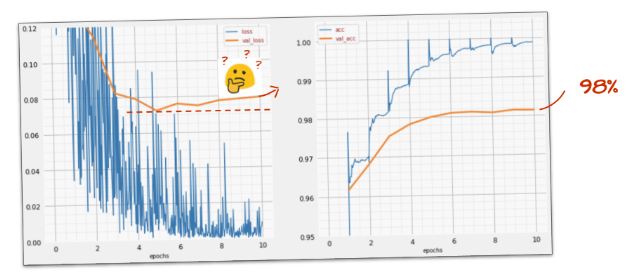dropout是深度学习中最古老的正则化技术之一。在每次训练迭代中，它以概率p（通常为25%到50%）从网络中丢弃随机神经元。实际上，神经元输出设置为0，最终的结果是这些神经元这次将不会参与损失计算，也不会得到权重的更新。在每次训练迭代中都会丢弃不同的神经元。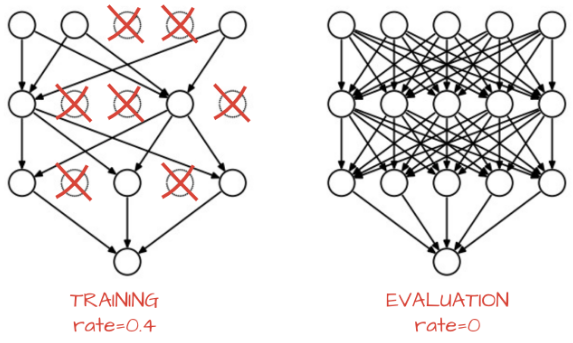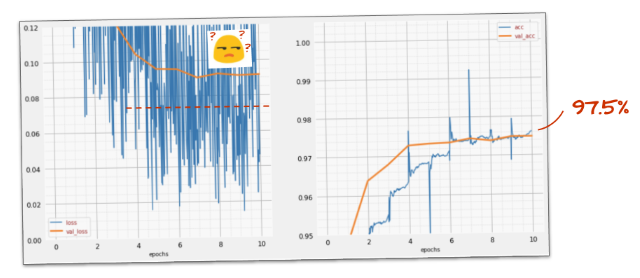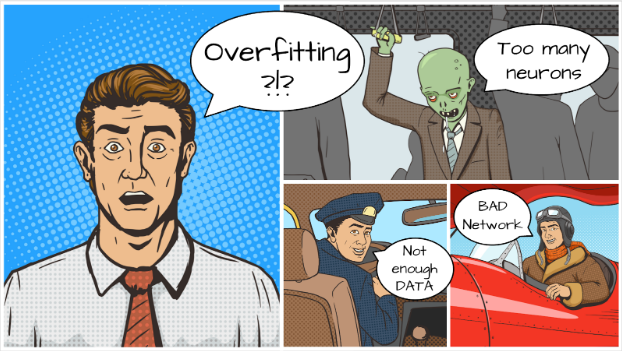keras_03_mnist_dense_lrdecay_dropout.ipynb

## 10. [INFO]卷积网络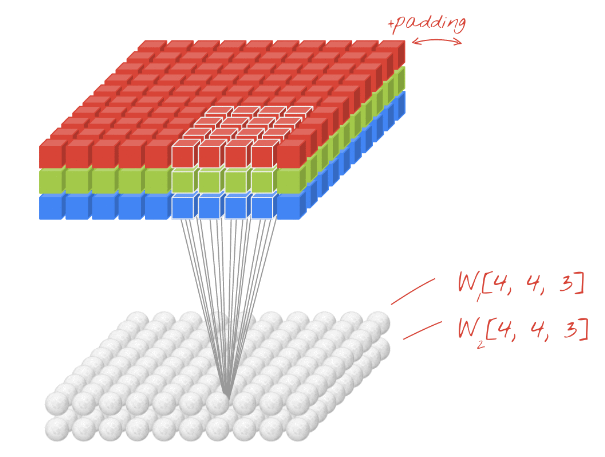1 2 3 4 5 6 7 8  model = tf.keras.Sequential([ tf.keras.layers.Reshape(input_shape=(28*28,), target_shape=(28, 28, 1)), tf.keras.layers.Conv2D(kernel_size=3, filters=12, activation='relu'), tf.keras.layers.Conv2D(kernel_size=6, filters=24, strides=2, activation='relu'), tf.keras.layers.Conv2D(kernel_size=6, filters=32, strides=2, activation='relu'), tf.keras.layers.Flatten(), tf.keras.layers.Dense(10, activation='softmax') ])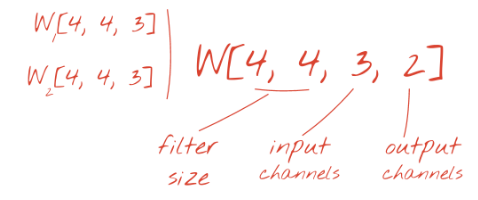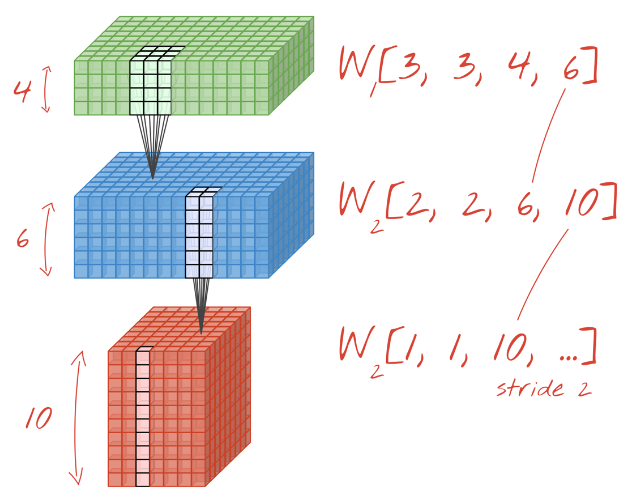• 交错卷积：如上所述的步长>1的滑动过滤器
• 最大池化：应用最大操作的滑动窗口（通常在2*2的小块上，每2个像素重复一次）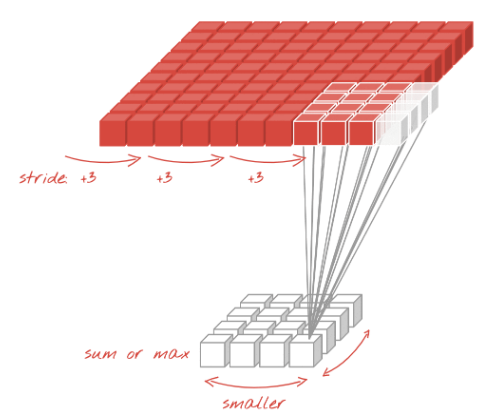“最大池化”可以帮助我们直观地理解卷积网络是如何工作的：如果假设在训练过程中，权重块演变为可以识别基本形状（水平和垂直线、曲线等）的过滤器，那么将有用的信息记下来的方法是保持通过这些层的输出，其形状能被最大强度的识别。实际上，在最大池化层中，神经元输出以2*2为一组进行处理，并且只保留最大值。## 11. 一个卷积网络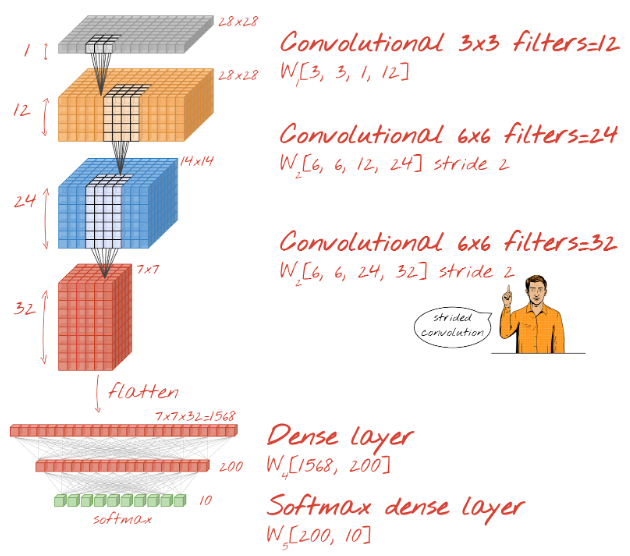1  tf.keras.layers.Reshape(input_shape=(28*28,), target_shape=(28, 28, 1))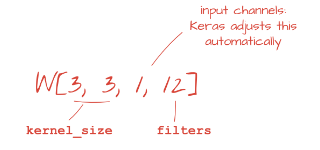1  tf.keras.layers.Conv2D(kernel_size=3, filters=12, padding='same', activation='relu') 

• “same”：用零填充，以便产生与输入相同的宽度/高度的输出
• “valid”：不填充，仅使用真实像素

 1  tf.keras.layers.Conv2D(kernel_size=6, filters=24, padding='same', activation='relu', strides=2) 

 1  tf.keras.layers.Flatten() 

 1  tf.keras.layers.Dense(200, activation='relu')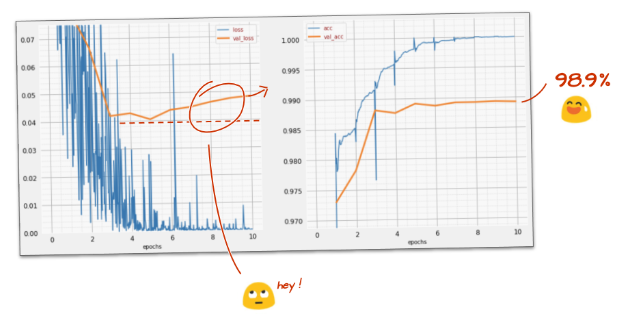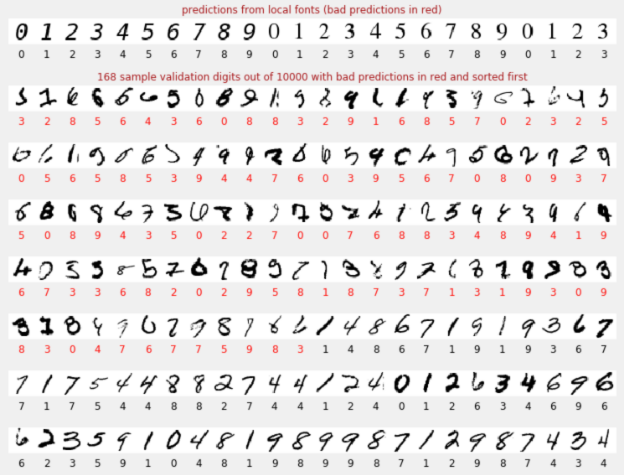keras_04_mnist_convolutional.ipynb

## 12. 再次dropout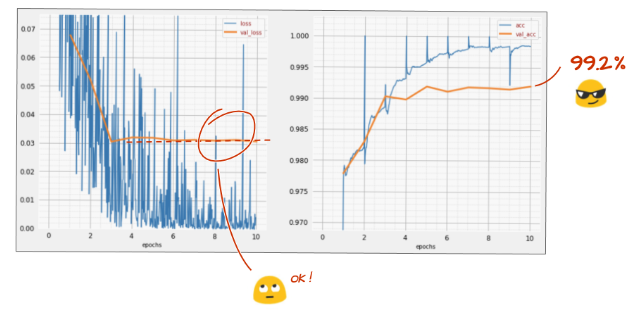## 13. 批量归一化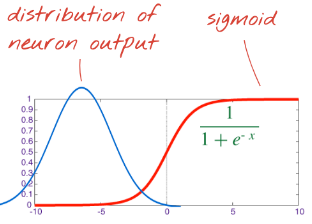sigmoid激活后，距离左侧太远，该神经元几乎总是输出0。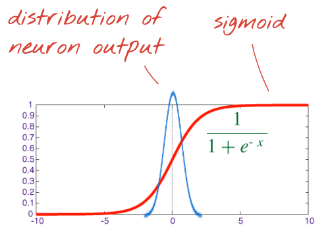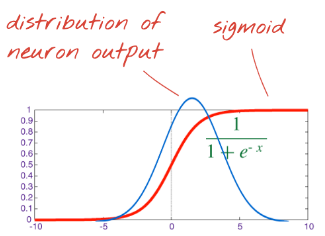$\alpha$ . normalized_out + $\beta$

 1  tf.keras.layers.BatchNormalization(scale=False, center=True) 

• 批量归一化介于层的输出与其激活函数之间。
• 如果你在批量归一化中使用center=True，则不需要在层中使用偏差，批量归一化的偏移量起着偏差的作用。
• 如果你使用的激活函数是比例不变的（即缩放后不会改变形态），则可以设置scale=False，Relu是比例不变的，sigmoid不是。

 1 2 3 4 5 6 7 8  # Modify each layer: remove the activation from the layer itself. # Set use_bias=False since batch norm will play the role of biases. tf.keras.layers.Conv2D(..., use_bias=False), # Batch norm goes between the layer and its activation. # The scale factor can be turned off for Relu activation. tf.keras.layers.BatchNormalization(scale=False, center=True), # Finish with the activation. tf.keras.layers.Activation('relu'),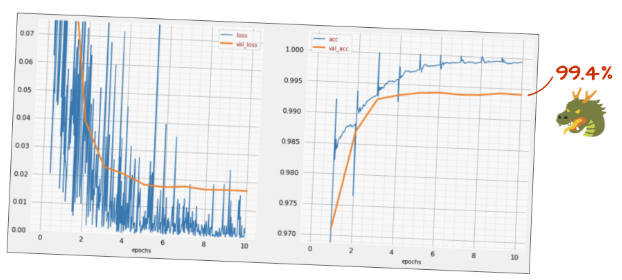• 批量归一化有助于神经网络收敛，通常可以让你训练得更快。
• 批量归一化是一个正则项，你通常可以减少使用dropout的量，甚至根本不使用dropout。

keras_05_mnist_batch_norm.ipynb

## 14. 在强大的硬件上进行云训练：AI平台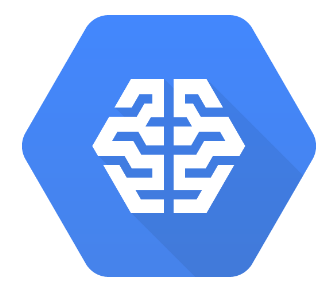2. 启用账单。
3. 安装GCP命令行工具。